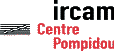Serveur © IRCAM - CENTRE POMPIDOU 1996-2005.Tous droits réservés pour tous pays. All rights reserved.

# Computation and Modeling of The Sound Radiation of An Upright Piano Using Modal Formalism and Integral Equations

Philippe Dérogis, René Caussé

ICA 95, Trondheim (Norvège), 1995

## Introduction

### Upright Piano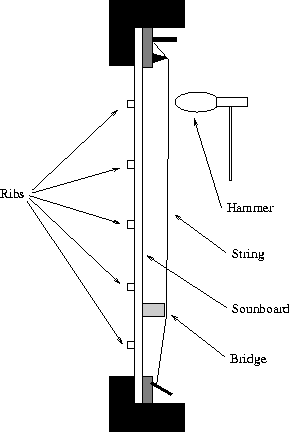## Modal Analysis

### Modal Formalism for general viscous damping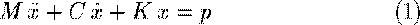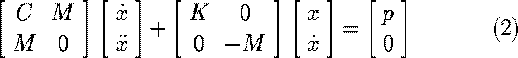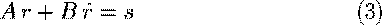## Eigenvalue Problem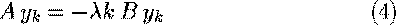where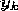can be writren as :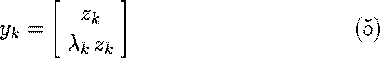where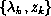are respectively the eigen values and the eigen vectors of equation(1)

## Modal parameters extraction

The response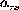at the point r to a sinusoidal excitation at the point s can be expressed using the eigen values and the eigen vectors of equation (1) :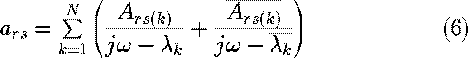where :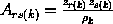## Computation of the displacement resulting from any excitation

The displacement x(t) resulting from an excitation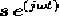can be computed using the formula :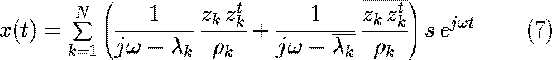where :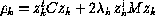## Mesasurements and Results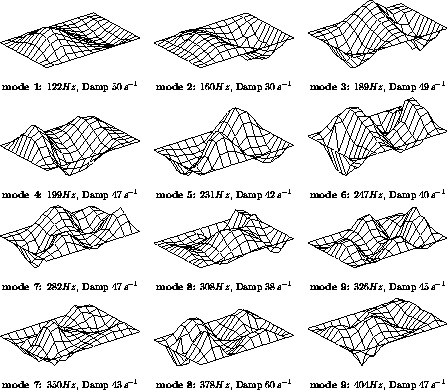Figure 1: Modes resulting from the curve-fiting of measurements

### Sound radiation of a baffled plate

The sound pressure p(r) and the acoustic velocity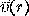resulting from the vibrations of a baffled plate moving with normal acceleration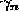are given by :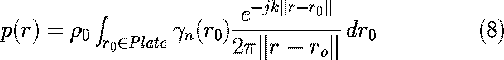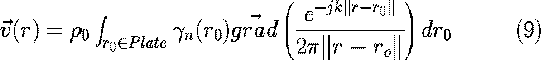## Sound pressure radiated by the first mode of the soundboard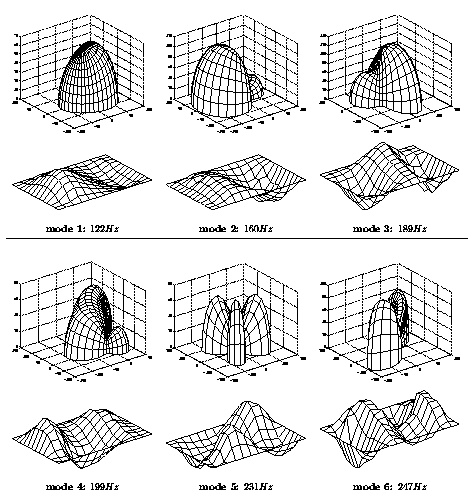## Normal Active Intensity

The active intensity is the power radiated per unit surface.
It is given by the formula :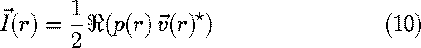It is intersting to calculate the z componant of the active intensity near the soundboard in order to know which regions produce the acoustic power, in particular :

• If it is positive, the energy travels from the soundboard to the acoustic field
• If it is negative, the energy travels from the acoustic field to the sounboard.

Computation of the normal Active Intensity at 5 centimeters of the soundboard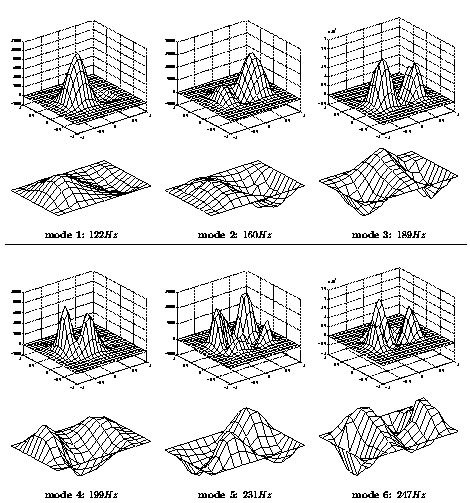## An example of a loop of active intensity

Active intensity field in a plane orthogonal to the plate for the mode 1 : 122Hz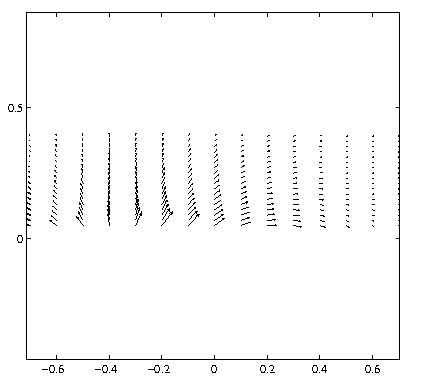The total power radiated by a source can be computed using :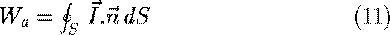## Power radiated by the soundboard versus frequency for several driving point

• Computation of the displacement of the soundboard using modal formalism
• Computation of the acoustic field
• Computation of the radiated power

## Results

Radiated power for several points excited by a 1N force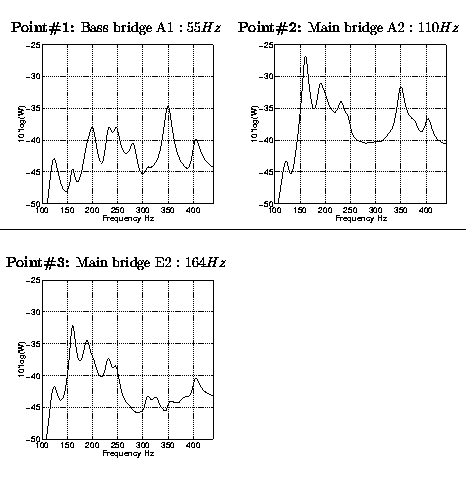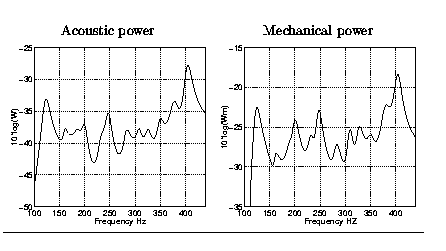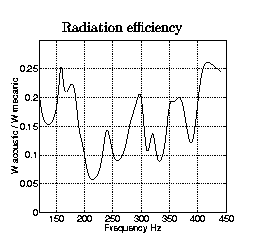Radiation efficiency of a soundboard having eigen values (frequency and damping) a factor 1.5 higher.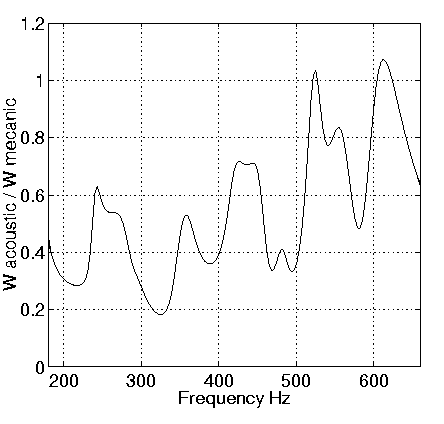## Conclusion

• The first modes of the soundboard look like the ones of an isotropic suported plate
• The pressure radiated by the modes depends strongly of the position of the observer
• The frequencies of the first modes are well below the coincidence frequency of these modes
• There are loops of active Intesity
• The acoustic power depends on the location of the excitation point